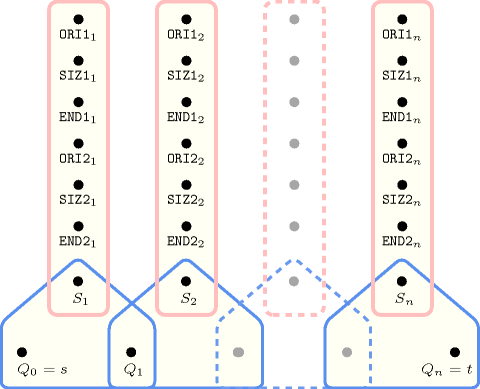## 5.408. two_orth_are_in_contact

Origin
Constraint

$\mathrm{𝚝𝚠𝚘}_\mathrm{𝚘𝚛𝚝𝚑}_\mathrm{𝚊𝚛𝚎}_\mathrm{𝚒𝚗}_\mathrm{𝚌𝚘𝚗𝚝𝚊𝚌𝚝}\left(\mathrm{𝙾𝚁𝚃𝙷𝙾𝚃𝙾𝙿𝙴}\mathtt{1},\mathrm{𝙾𝚁𝚃𝙷𝙾𝚃𝙾𝙿𝙴}\mathtt{2}\right)$

Type
 $\mathrm{𝙾𝚁𝚃𝙷𝙾𝚃𝙾𝙿𝙴}$ $\mathrm{𝚌𝚘𝚕𝚕𝚎𝚌𝚝𝚒𝚘𝚗}\left(\mathrm{𝚘𝚛𝚒}-\mathrm{𝚍𝚟𝚊𝚛},\mathrm{𝚜𝚒𝚣}-\mathrm{𝚍𝚟𝚊𝚛},\mathrm{𝚎𝚗𝚍}-\mathrm{𝚍𝚟𝚊𝚛}\right)$
Arguments
 $\mathrm{𝙾𝚁𝚃𝙷𝙾𝚃𝙾𝙿𝙴}\mathtt{1}$ $\mathrm{𝙾𝚁𝚃𝙷𝙾𝚃𝙾𝙿𝙴}$ $\mathrm{𝙾𝚁𝚃𝙷𝙾𝚃𝙾𝙿𝙴}\mathtt{2}$ $\mathrm{𝙾𝚁𝚃𝙷𝙾𝚃𝙾𝙿𝙴}$
Restrictions
 $|\mathrm{𝙾𝚁𝚃𝙷𝙾𝚃𝙾𝙿𝙴}|>0$ $\mathrm{𝚛𝚎𝚚𝚞𝚒𝚛𝚎}_\mathrm{𝚊𝚝}_\mathrm{𝚕𝚎𝚊𝚜𝚝}$$\left(2,\mathrm{𝙾𝚁𝚃𝙷𝙾𝚃𝙾𝙿𝙴},\left[\mathrm{𝚘𝚛𝚒},\mathrm{𝚜𝚒𝚣},\mathrm{𝚎𝚗𝚍}\right]\right)$ $\mathrm{𝙾𝚁𝚃𝙷𝙾𝚃𝙾𝙿𝙴}.\mathrm{𝚜𝚒𝚣}>0$ $\mathrm{𝙾𝚁𝚃𝙷𝙾𝚃𝙾𝙿𝙴}.\mathrm{𝚘𝚛𝚒}\le \mathrm{𝙾𝚁𝚃𝙷𝙾𝚃𝙾𝙿𝙴}.\mathrm{𝚎𝚗𝚍}$ $|\mathrm{𝙾𝚁𝚃𝙷𝙾𝚃𝙾𝙿𝙴}\mathtt{1}|=|\mathrm{𝙾𝚁𝚃𝙷𝙾𝚃𝙾𝙿𝙴}\mathtt{2}|$ $\mathrm{𝚘𝚛𝚝𝚑}_\mathrm{𝚕𝚒𝚗𝚔}_\mathrm{𝚘𝚛𝚒}_\mathrm{𝚜𝚒𝚣}_\mathrm{𝚎𝚗𝚍}$$\left(\mathrm{𝙾𝚁𝚃𝙷𝙾𝚃𝙾𝙿𝙴}\mathtt{1}\right)$ $\mathrm{𝚘𝚛𝚝𝚑}_\mathrm{𝚕𝚒𝚗𝚔}_\mathrm{𝚘𝚛𝚒}_\mathrm{𝚜𝚒𝚣}_\mathrm{𝚎𝚗𝚍}$$\left(\mathrm{𝙾𝚁𝚃𝙷𝙾𝚃𝙾𝙿𝙴}\mathtt{2}\right)$
Purpose

Enforce the following conditions on two orthotopes ${O}_{1}$ and ${O}_{2}$:

• For all dimensions $i$, except one dimension, the projections of ${O}_{1}$ and ${O}_{2}$ onto $i$ have a non-empty intersection.

• For all dimensions $i$, the distance between the projections of ${O}_{1}$ and ${O}_{2}$ onto $i$ is equal to 0.

Example
$\left(\begin{array}{c}〈\mathrm{𝚘𝚛𝚒}-1\mathrm{𝚜𝚒𝚣}-3\mathrm{𝚎𝚗𝚍}-4,\mathrm{𝚘𝚛𝚒}-5\mathrm{𝚜𝚒𝚣}-2\mathrm{𝚎𝚗𝚍}-7〉,\hfill \\ 〈\mathrm{𝚘𝚛𝚒}-3\mathrm{𝚜𝚒𝚣}-2\mathrm{𝚎𝚗𝚍}-5,\mathrm{𝚘𝚛𝚒}-2\mathrm{𝚜𝚒𝚣}-3\mathrm{𝚎𝚗𝚍}-5〉\hfill \end{array}\right)$

Figure 5.408.1 shows the two rectangles of the example. The $\mathrm{𝚝𝚠𝚘}_\mathrm{𝚘𝚛𝚝𝚑}_\mathrm{𝚊𝚛𝚎}_\mathrm{𝚒𝚗}_\mathrm{𝚌𝚘𝚗𝚝𝚊𝚌𝚝}$ constraint holds since the two rectangles are in contact: the contact is depicted by a pink line-segment.

##### Figure 5.408.1. The two rectangles that are in contact of the Example slot where the contact is shown in pink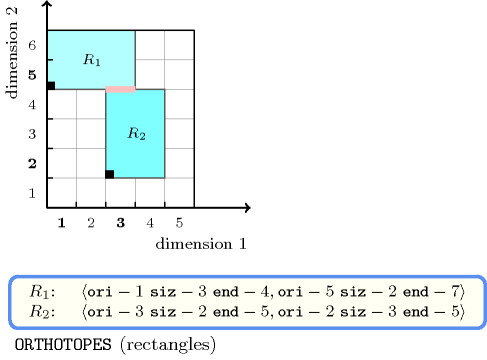Typical
$|\mathrm{𝙾𝚁𝚃𝙷𝙾𝚃𝙾𝙿𝙴}|>1$
Symmetries
• Arguments are permutable w.r.t. permutation $\left(\mathrm{𝙾𝚁𝚃𝙷𝙾𝚃𝙾𝙿𝙴}\mathtt{1},\mathrm{𝙾𝚁𝚃𝙷𝙾𝚃𝙾𝙿𝙴}\mathtt{2}\right)$.

• Items of $\mathrm{𝙾𝚁𝚃𝙷𝙾𝚃𝙾𝙿𝙴}\mathtt{1}$ and $\mathrm{𝙾𝚁𝚃𝙷𝙾𝚃𝙾𝙿𝙴}\mathtt{2}$ are permutable (same permutation used).

Used in
Keywords
Arc input(s)

$\mathrm{𝙾𝚁𝚃𝙷𝙾𝚃𝙾𝙿𝙴}\mathtt{1}$ $\mathrm{𝙾𝚁𝚃𝙷𝙾𝚃𝙾𝙿𝙴}\mathtt{2}$

Arc generator
$\mathrm{𝑃𝑅𝑂𝐷𝑈𝐶𝑇}$$\left(=\right)↦\mathrm{𝚌𝚘𝚕𝚕𝚎𝚌𝚝𝚒𝚘𝚗}\left(\mathrm{𝚘𝚛𝚝𝚑𝚘𝚝𝚘𝚙𝚎}\mathtt{1},\mathrm{𝚘𝚛𝚝𝚑𝚘𝚝𝚘𝚙𝚎}\mathtt{2}\right)$

Arc arity
Arc constraint(s)
 $•\mathrm{𝚘𝚛𝚝𝚑𝚘𝚝𝚘𝚙𝚎}\mathtt{1}.\mathrm{𝚎𝚗𝚍}>\mathrm{𝚘𝚛𝚝𝚑𝚘𝚝𝚘𝚙𝚎}\mathtt{2}.\mathrm{𝚘𝚛𝚒}$ $•\mathrm{𝚘𝚛𝚝𝚑𝚘𝚝𝚘𝚙𝚎}\mathtt{2}.\mathrm{𝚎𝚗𝚍}>\mathrm{𝚘𝚛𝚝𝚑𝚘𝚝𝚘𝚙𝚎}\mathtt{1}.\mathrm{𝚘𝚛𝚒}$
Graph property(ies)
$\mathrm{𝐍𝐀𝐑𝐂}$$=|\mathrm{𝙾𝚁𝚃𝙷𝙾𝚃𝙾𝙿𝙴}\mathtt{1}|-1$

Arc input(s)

$\mathrm{𝙾𝚁𝚃𝙷𝙾𝚃𝙾𝙿𝙴}\mathtt{1}$ $\mathrm{𝙾𝚁𝚃𝙷𝙾𝚃𝙾𝙿𝙴}\mathtt{2}$

Arc generator
$\mathrm{𝑃𝑅𝑂𝐷𝑈𝐶𝑇}$$\left(=\right)↦\mathrm{𝚌𝚘𝚕𝚕𝚎𝚌𝚝𝚒𝚘𝚗}\left(\mathrm{𝚘𝚛𝚝𝚑𝚘𝚝𝚘𝚙𝚎}\mathtt{1},\mathrm{𝚘𝚛𝚝𝚑𝚘𝚝𝚘𝚙𝚎}\mathtt{2}\right)$

Arc arity
Arc constraint(s)
$\mathrm{𝚖𝚊𝚡}\left(\begin{array}{c}0,\begin{array}{c}\mathrm{𝚖𝚊𝚡}\left(\mathrm{𝚘𝚛𝚝𝚑𝚘𝚝𝚘𝚙𝚎}\mathtt{1}.\mathrm{𝚘𝚛𝚒},\mathrm{𝚘𝚛𝚝𝚑𝚘𝚝𝚘𝚙𝚎}\mathtt{2}.\mathrm{𝚘𝚛𝚒}\right)-\hfill \\ \mathrm{𝚖𝚒𝚗}\left(\mathrm{𝚘𝚛𝚝𝚑𝚘𝚝𝚘𝚙𝚎}\mathtt{1}.\mathrm{𝚎𝚗𝚍},\mathrm{𝚘𝚛𝚝𝚑𝚘𝚝𝚘𝚙𝚎}\mathtt{2}.\mathrm{𝚎𝚗𝚍}\right)\hfill \end{array}\hfill \end{array}\right)=0$
Graph property(ies)
$\mathrm{𝐍𝐀𝐑𝐂}$$=|\mathrm{𝙾𝚁𝚃𝙷𝙾𝚃𝙾𝙿𝙴}\mathtt{1}|$

Graph model

Parts (A) and (B) of Figure 5.408.2 respectively show the initial and final graph associated with the first graph constraint of the Example slot. Since we use the $\mathrm{𝐍𝐀𝐑𝐂}$ graph property, the unique arc of the final graph is stressed in bold. It corresponds to the fact that the projection onto dimension 1 of the two rectangles of the example overlap.

##### Figure 5.408.2. Initial and final graph of the $\mathrm{𝚝𝚠𝚘}_\mathrm{𝚘𝚛𝚝𝚑}_\mathrm{𝚊𝚛𝚎}_\mathrm{𝚒𝚗}_\mathrm{𝚌𝚘𝚗𝚝𝚊𝚌𝚝}$ constraint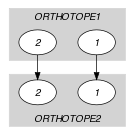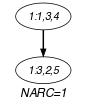(a) (b)
Signature

Consider the second graph constraint. Since we use the arc generator $\mathrm{𝑃𝑅𝑂𝐷𝑈𝐶𝑇}\left(=\right)$ on the collections $\mathrm{𝙾𝚁𝚃𝙷𝙾𝚃𝙾𝙿𝙴}\mathtt{1}$ and $\mathrm{𝙾𝚁𝚃𝙷𝙾𝚃𝙾𝙿𝙴}\mathtt{2}$, and because of the restriction $|\mathrm{𝙾𝚁𝚃𝙷𝙾𝚃𝙾𝙿𝙴}\mathtt{1}|=|\mathrm{𝙾𝚁𝚃𝙷𝙾𝚃𝙾𝙿𝙴}\mathtt{2}|$, the maximum number of arcs of the corresponding final graph is equal to $|\mathrm{𝙾𝚁𝚃𝙷𝙾𝚃𝙾𝙿𝙴}\mathtt{1}|$. Therefore we can rewrite the graph property $\mathrm{𝐍𝐀𝐑𝐂}=|\mathrm{𝙾𝚁𝚃𝙷𝙾𝚃𝙾𝙿𝙴}\mathtt{1}|$ to $\mathrm{𝐍𝐀𝐑𝐂}\ge |\mathrm{𝙾𝚁𝚃𝙷𝙾𝚃𝙾𝙿𝙴}\mathtt{1}|$ and simplify $\underline{\overline{\mathrm{𝐍𝐀𝐑𝐂}}}$ to $\overline{\mathrm{𝐍𝐀𝐑𝐂}}$.

Automaton

Figure 5.408.3 depicts the automaton associated with the $\mathrm{𝚝𝚠𝚘}_\mathrm{𝚘𝚛𝚝𝚑}_\mathrm{𝚊𝚛𝚎}_\mathrm{𝚒𝚗}_\mathrm{𝚌𝚘𝚗𝚝𝚊𝚌𝚝}$ constraint. Let $\mathrm{𝙾𝚁𝙸}{\mathtt{1}}_{i}$, $\mathrm{𝚂𝙸𝚉}{\mathtt{1}}_{i}$ and $\mathrm{𝙴𝙽𝙳}{\mathtt{1}}_{i}$ respectively be the $\mathrm{𝚘𝚛𝚒}$, the $\mathrm{𝚜𝚒𝚣}$ and the $\mathrm{𝚎𝚗𝚍}$ attributes of the ${i}^{th}$ item of the $\mathrm{𝙾𝚁𝚃𝙷𝙾𝚃𝙾𝙿𝙴}\mathtt{1}$ collection. Let $\mathrm{𝙾𝚁𝙸}{\mathtt{2}}_{i}$, $\mathrm{𝚂𝙸𝚉}{\mathtt{2}}_{i}$ and $\mathrm{𝙴𝙽𝙳}{\mathtt{2}}_{i}$ respectively be the $\mathrm{𝚘𝚛𝚒}$, the $\mathrm{𝚜𝚒𝚣}$ and the $\mathrm{𝚎𝚗𝚍}$ attributes of the ${i}^{th}$ item of the $\mathrm{𝙾𝚁𝚃𝙷𝙾𝚃𝙾𝙿𝙴}\mathtt{2}$ collection. To each sextuple $\left(\mathrm{𝙾𝚁𝙸}{\mathtt{1}}_{i},\mathrm{𝚂𝙸𝚉}{\mathtt{1}}_{i},\mathrm{𝙴𝙽𝙳}{\mathtt{1}}_{i},\mathrm{𝙾𝚁𝙸}{\mathtt{2}}_{i},\mathrm{𝚂𝙸𝚉}{\mathtt{2}}_{i},\mathrm{𝙴𝙽𝙳}{\mathtt{2}}_{i}\right)$ corresponds a signature variable ${S}_{i}$, which takes its value in $\left\{0,1,2\right\}$, as well as the following signature constraint:

$\left(\left(\mathrm{𝚂𝙸𝚉}{\mathtt{1}}_{i}>0\right)\wedge \left(\mathrm{𝚂𝙸𝚉}{\mathtt{2}}_{i}>0\right)\wedge \left(\mathrm{𝙴𝙽𝙳}{\mathtt{1}}_{i}>\mathrm{𝙾𝚁𝙸}{\mathtt{2}}_{i}\right)\wedge \left(\mathrm{𝙴𝙽𝙳}{\mathtt{2}}_{i}>\mathrm{𝙾𝚁𝙸}{\mathtt{1}}_{i}\right)\right)⇔{S}_{i}=0$

$\left(\left(\mathrm{𝚂𝙸𝚉}{\mathtt{1}}_{i}>0\right)\wedge \left(\mathrm{𝚂𝙸𝚉}{\mathtt{2}}_{i}>0\right)\wedge \left(\mathrm{𝙴𝙽𝙳}{\mathtt{1}}_{i}=\mathrm{𝙾𝚁𝙸}{\mathtt{2}}_{i}\vee \mathrm{𝙴𝙽𝙳}{\mathtt{2}}_{i}=\mathrm{𝙾𝚁𝙸}{\mathtt{1}}_{i}\right)\right)⇔{S}_{i}=1$.

##### Figure 5.408.3. Automaton of the $\mathrm{𝚝𝚠𝚘}_\mathrm{𝚘𝚛𝚝𝚑}_\mathrm{𝚊𝚛𝚎}_\mathrm{𝚒𝚗}_\mathrm{𝚌𝚘𝚗𝚝𝚊𝚌𝚝}$ constraint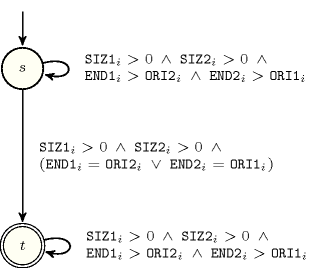##### Figure 5.408.4. Hypergraph of the reformulation corresponding to the automaton of the $\mathrm{𝚝𝚠𝚘}_\mathrm{𝚘𝚛𝚝𝚑}_\mathrm{𝚊𝚛𝚎}_\mathrm{𝚒𝚗}_\mathrm{𝚌𝚘𝚗𝚝𝚊𝚌𝚝}$ constraint